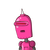# If diagonal of a rectangle ABCD intersect at point o,if AC=8 then OC=? pls reply me fast​

If diagonal of a rectangle ABCD intersect at point o,if AC=8 then OC=? pls reply me fast​

### 1 thought on “If diagonal of a rectangle ABCD intersect at point o,if AC=8 then OC=? pls reply me fast​”

1.Step-by-step explanation:

Diagonals of a rectangle are congruent.

So, AC=BD=8 cm

Also, rectangle is a parallelogram so, the diagonals bisect each other.

Thus, BO=OD=4 cm